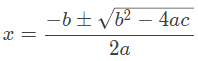Search

# Quadratic Equation using Android Application.

Updated: Nov 18, 2020

A Quadratic Equation is usually written ax2 + bx + c = 0, where x is an unknown variable and a,b,c are numerical coefficients Here, a ≠ 0 because if it equals to zero then the equation will not remain quadratic anymore and it will become a linear equation, such as bx+c=0.